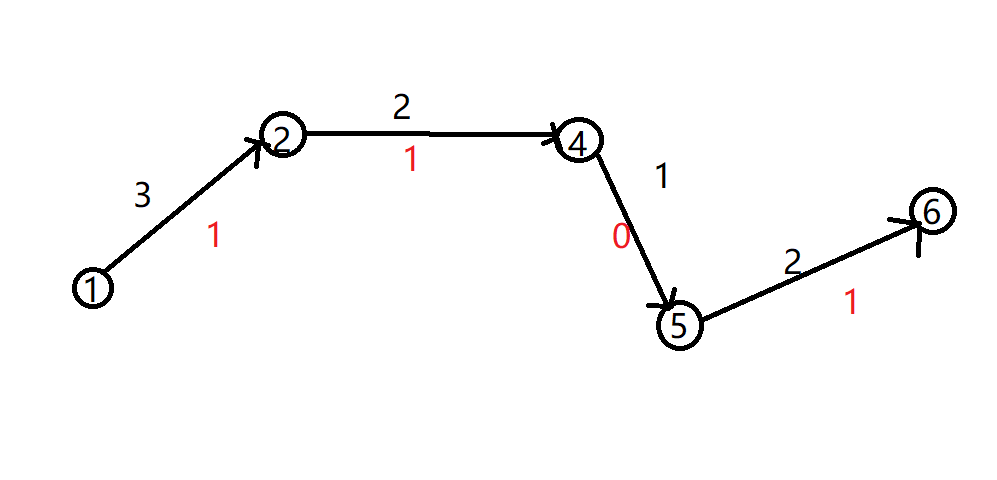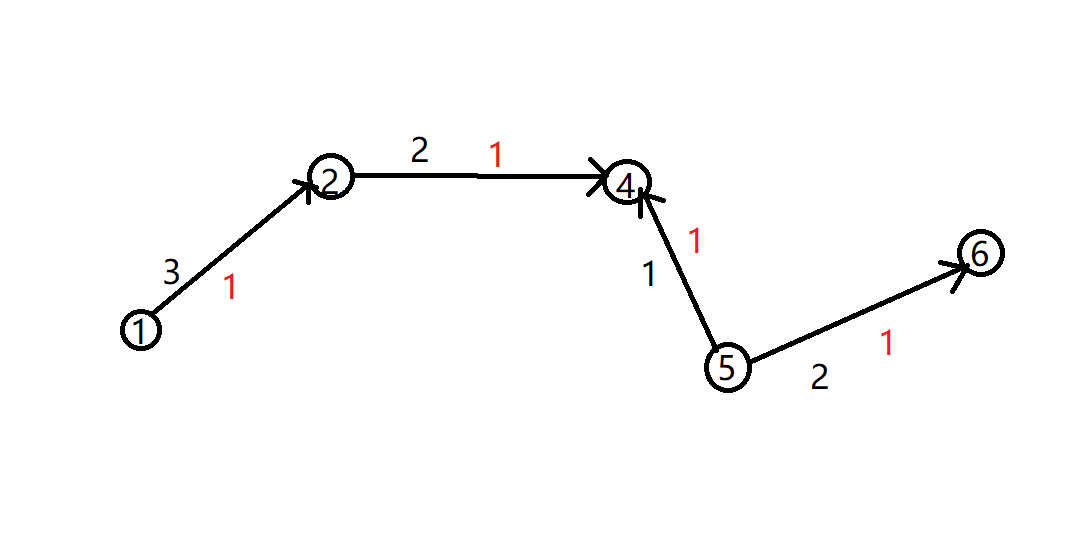# 最大流算法思想和理论的简单理解

## 1.定义

1.对于一个有向的网络$G=(N, A, C)$,其中的$c_{i,j}$表示弧$(i,j)\in A$的容量，并设$s,t$为发点和收点，令
$$x_{i,j}=通过弧(i,j)的流量$$
2.对于一个满足流量限制的流量称之为可行流，其中限制条件为
(1)守恒方程(对于非收发点，流入流量等于流出流量)
\sum_j x_{i,j}-\sum_j x_{j,i}=\left\{ \begin{aligned} +v,\,\,\, i =s \\ 0,\,\,\, i \neq s,t \\ -v,\,\,\, i =t \end{aligned} \right.

(2)$0\leq x_{i,j} \leq c_{i,j}$
3.设P是G中从s到t的无向路，若弧$(i,j)$的方向是从s到t，那么称之为前向弧，否则为后向弧。
4.如果对于G的一个无向路P，有前向弧$(i,j)$，$x_{i,j} < c_{i,j}$。有后向弧$(i,j)$，$x_{i, j}>0$则称P为增广路。

## 2.算法思想

1.增广路上都是前向弧。2.增广路上有后向弧。## 3.算法的理论证明

1.增广路定理：一个可行流是最大流当且仅当不存在关于它从s到t的增广路。

$$v\leq C(S,T)$$

$$v=\sum_{i\in S}\sum_{j\in T}x_{i,j}=\sum_{i\in S}\sum_{j\in T}c_{i,j}=C(S,T)$$

2.最大流最小割定理：一个$(s,t)$流的最大值为$(s,t)$割的最小容量。

posted @ 2018-06-29 23:36  zprhhs  阅读(1348)  评论(0编辑  收藏  举报